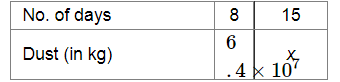# In 8 days, the earth picks up

Question:

In 8 days, the earth picks up (6.4 × 107) kg of dust from the atmosphere. How much dust will it pick up in 15 days?

Solution:

Let x kg be the required amount of dust. Then, we have:Clearly, more amount of dust will be collected in more number of days.

So, this is a case of direct proportion.

Now, $\frac{8}{6.4 \times 10^{7}}=\frac{15}{x}$

$\Rightarrow x=\frac{15 \times 6.4 \times 10^{7}}{8}$

$\Rightarrow x=12 \times 10^{7}$

Therefore, 12,00,00,000 kg of dust will be picked up in 15 days.